## How to add or subtract days, months, and years to date in Excel?

This article provides 7 solutions to add or subtract days, weeks, months, years, or a combination of years/months/days to a date in Excel. Video Demo

#### Add or subtract days to date with formula

You may have noticed that dates are 5-digit numbers in Excel. Therefore, you can add or subtract days as easy as adding or minus the number of days in Excel.

= date + number of days

1. Select a blank cell you will place the calculating result, type the formula =A2+10, and press the Enter key.
Note: For subtracting 10 days from the date, please use this formula =A2–10.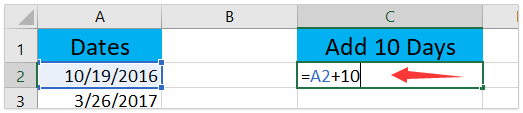2. If you need to apply this formula to other cells, please drag the AutoFill handle of the formula cell to these cells as you need.

Now you have added or subtracted the same number of days from these dates in bulk. See screenshot: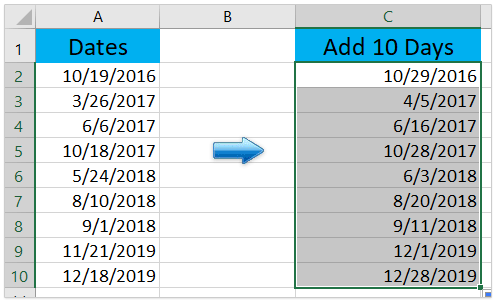#### Easily add/subtract days, weeks, Months, or Years to date in Excel

Difficult and tedious to remember long complicated formulas in Excel? Kutools for Excel’s Formula Helper lists most common-used formulas to help you quickly calculate and solve problems in Excel, says Add years / months / weeks / days to date, Add hours / minutes / seconds to date, Sum absolute values, Find most common value, etc.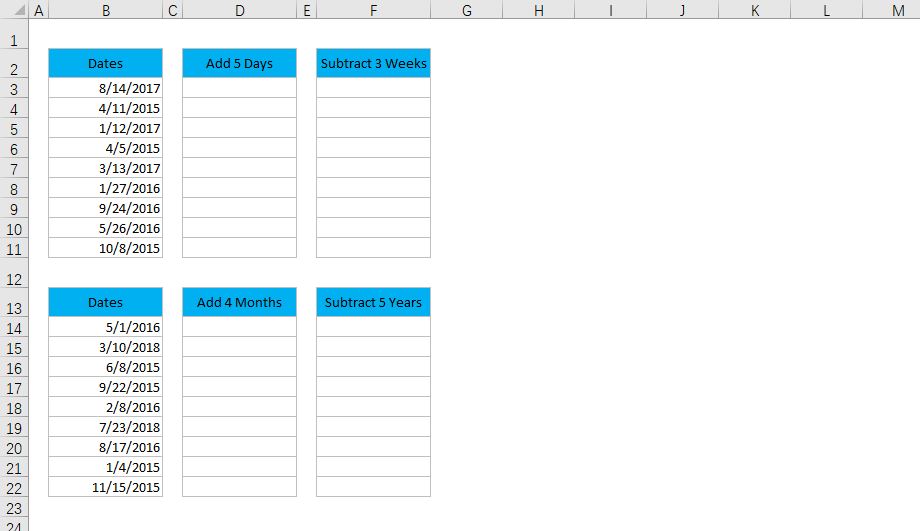Kutools for Excel - Supercharge Excel with over 300 essential tools. Enjoy a full-featured 30-day FREE trial with no credit card required! Get It Now

#### Add or subtract days to multiple dates without formula

Comparing to formulas, some Excel users prefer to add or subtract days directly without formulas. Here I will guide you to add or subtract the same number of days from multiple dates with Paste Special feature in Excel.

1. Type the number of days you will add or subtract to dates in a blank cell, says 10, and then copy it. See screenshot: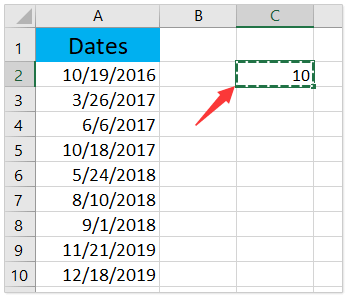2. Select the dates you will add or subtract days, right click, and select Paste Special > Paste Special in the context menu. See screenshot: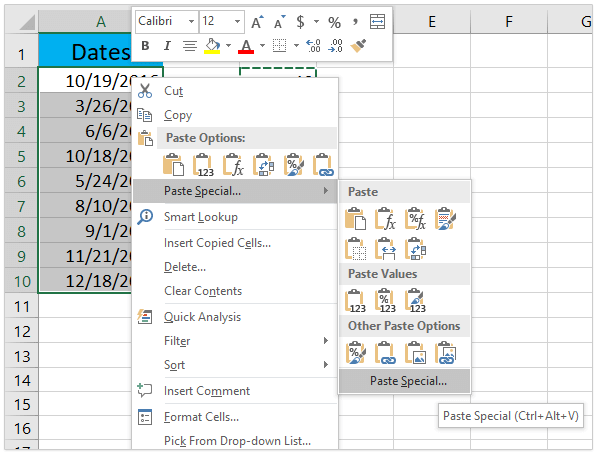3. In the Paste Special dialog, please check Add or Subtract options as you need, and click the OK button. See screenshot: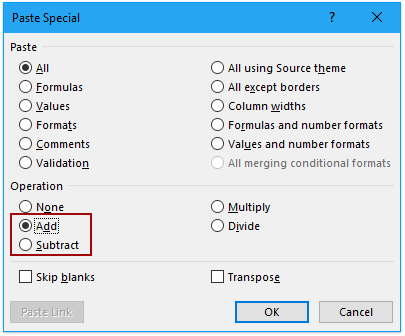4. Now the number is added or subtracted to the dates, and the dates display as 5-digit numbers. Keep these 5-digit numbers selected, and click Home > Number Format box > Short Date to convert them to dates again.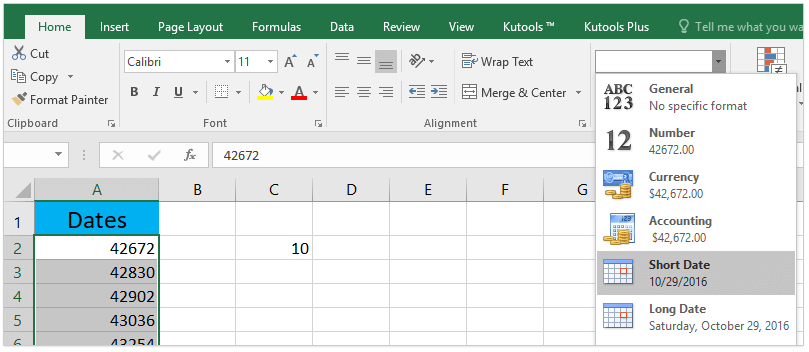Now you will see the specified number of days is added or subtracted from the specified range of dates in bulk without formula. See screenshot: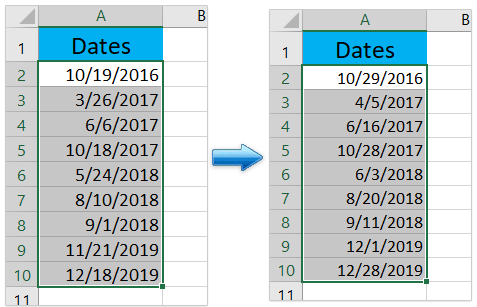#### Add or subtract weeks to date in Excel

You can also apply formula to add or subtract the specified number of weeks from a date in Excel. Please do as follows:

= date + 7 * number of weeks

1. Select the blank cell you will place the calculating result, type the formula =A2+4*7, and press the Enter key.
Note: For subtracting 4 weeks from the date, please use this formula =A2-4*7.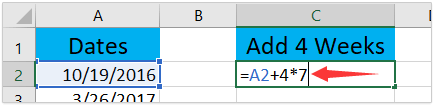Kutools for Excel### Stand out from the Crowd

300 Handy Tools
Solve 80% Problems in Excel

Say goodbye to terrible VBA and formulas!

2. If you need to add or subtract weeks from other dates, please drag the AutoFill handle of the formula cell as you need.

Now you have added or subtracted the same number of weeks from multiple dates in bulk. See screenshot: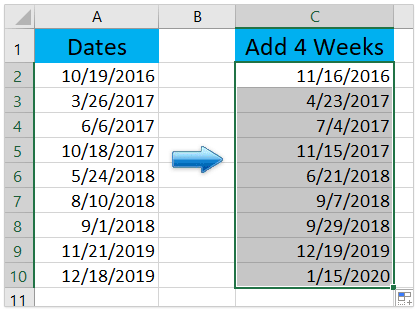#### Add or subtract months to date in Excel

For adding or subtracting months to dates, it’s not fit to add or subtract 30 from the dates because months contain different number of days in a year, some contain 30 days, some contain 31 days, while some contain 28 or 29 days. Therefore, we need to apply the EDATE function to deal with this situation.

= EDATE(date, number of months)

1. Select the blank cell you will place the calculating result, type the formula =EDATE(A2,3), and drag this formula cell’s AutoFill handle to apply this formula to other cells as you need.
Note: To subtracting 3 months from the date, please use this formula =EDATE(A2,-3).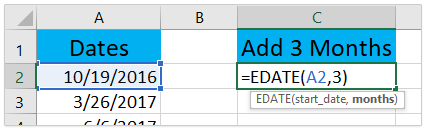2. As you see, the EDATE function will return 5-digit numbers. Please keep these 5-digit numbers selected, and click Home > Number Format box > Short Date to convert them to dates back. See screenshot: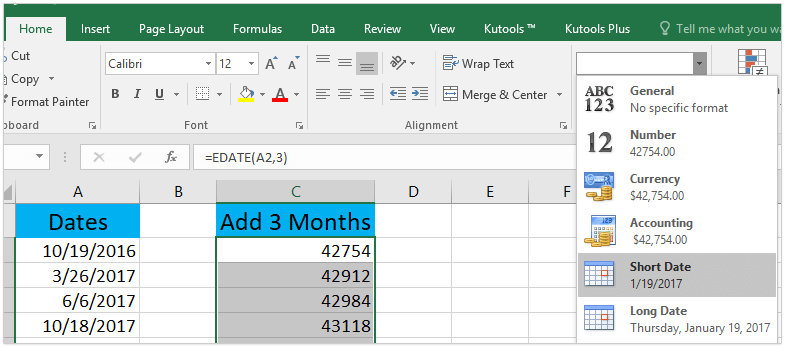Now you will see it has added or subtracted the same number of months to the dates in bulk. See screenshot: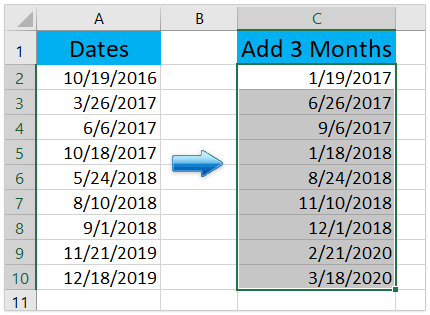#### Add or subtract years to date in Excel

For example, you will add 6 years to a batch of dates in Excel, you can do as follows:

= DATE(YEAR(date) + number of years, MONTH(date),DAY(date))

1. Select the blank cell you will place the calculating result, type the formula =DATE(YEAR(A2)+6,MONTH(A2),DAY(A2)), and press the Enter key.
Note: To subtract 6 years from the date, please apply this formula =DATE(YEAR(A2)-6,MONTH(A2),DAY(A2)).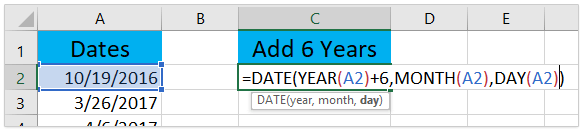2. If needed, drag the formula cell’s AutoFill handle to apply this formula to other cells as you need.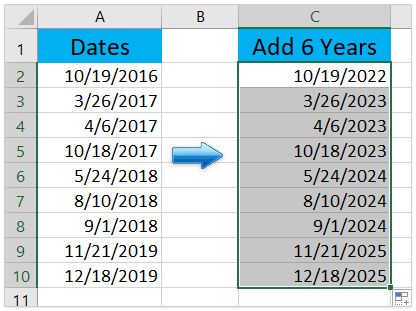#### Add or subtract a combination of years, month, and days to date in Excel

In some cases, you may need to add or subtract years, months, and days at the same time, says add 3 years 5 months and 15 days. In this case, you can apply the DATE function to solve the problem.

= DATE(YEAR(date) + number of years, MONTH(date) + number of months, DAY(date) + number of days)

1. Select the blank cell you will place the calculating result, type the formula =DATE(YEAR(A2)+3,MONTH(A2)+5,DAY(A2)+15), and press the Enter key. See screenshot:
Note: To subtract the combination of years, months, and days together, please use this formula =DATE(YEAR(A2)-3,MONTH(A2)-5,DAY(A2)-15).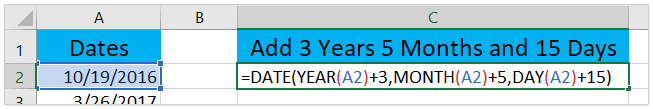2. If needed, please drag this formula cell’s AutoFill handle to apply this formula to other cells.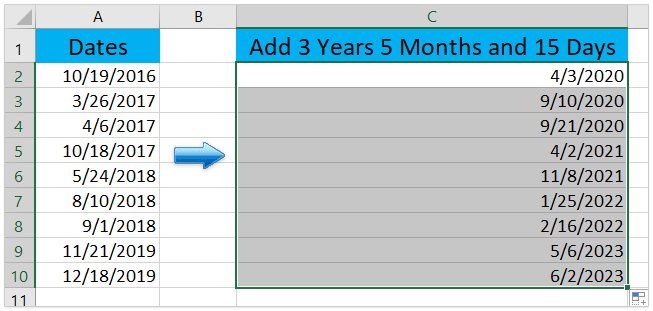#### Add or subtract days, weeks, months, or years to date with Kutools for Excel

If you have Kutools for Excel installed, you will find its Formula Helper lists most-commonly-used formulas to help you easily calculate in Excel, including adding days, weeks, months, or years to date. Please do as follows:

Kutools for Excel - Supercharge Excel with over 300 essential tools. Enjoy a full-featured 30-day FREE trial with no credit card required! Get It Now

1. Select the cell you will put calculating result in, and click Kutools > Formula Helper > Add years to date.
Tip: Please select the suitable formula from the Formula Helper drop down list based on your needs. For example, select Add weeks to date for adding/subtracting specified weeks to a date.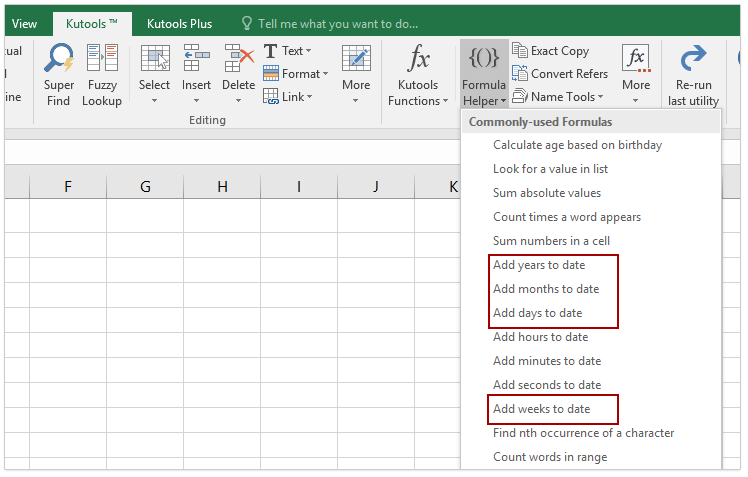2. In the opening Formula Helper dialog box, specify the date cell address in the Date Time box, and type the number of years you will add in the Number box.
Tip: In our case, we will add 5 year from the date, therefore we type 5 into the Number box. If you need to subtract some years, for example 3 years, please type -3 into the Number box.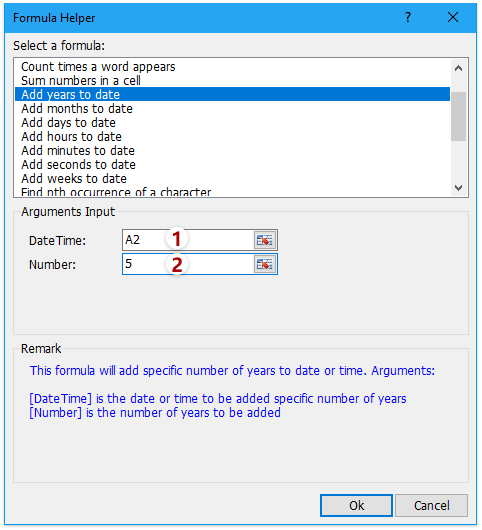3. Click the Ok button to apply the formula of Add years to date. Please drag the Fill Handle to apply this formula to other range if necessary.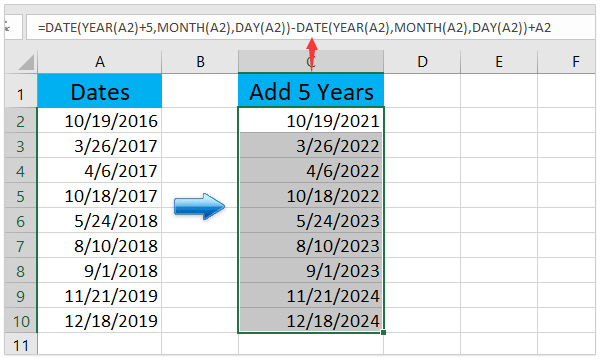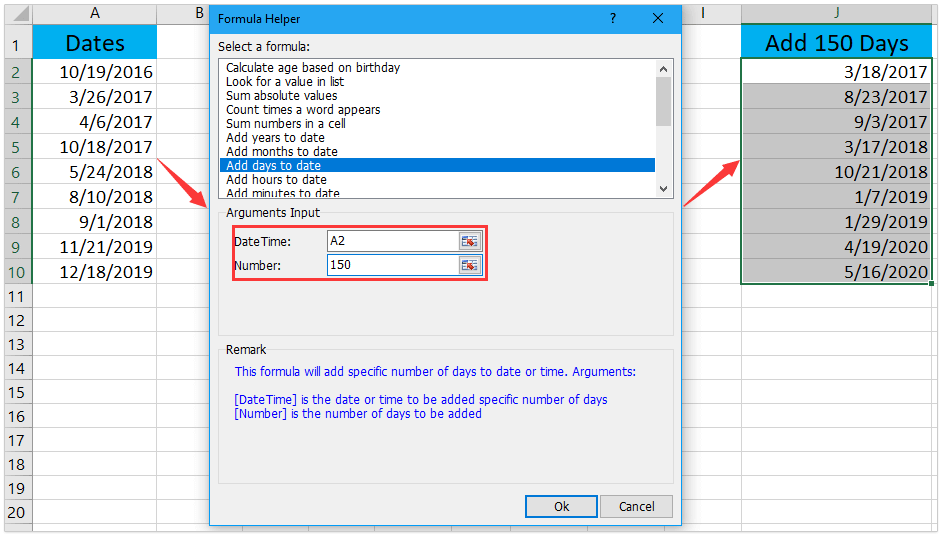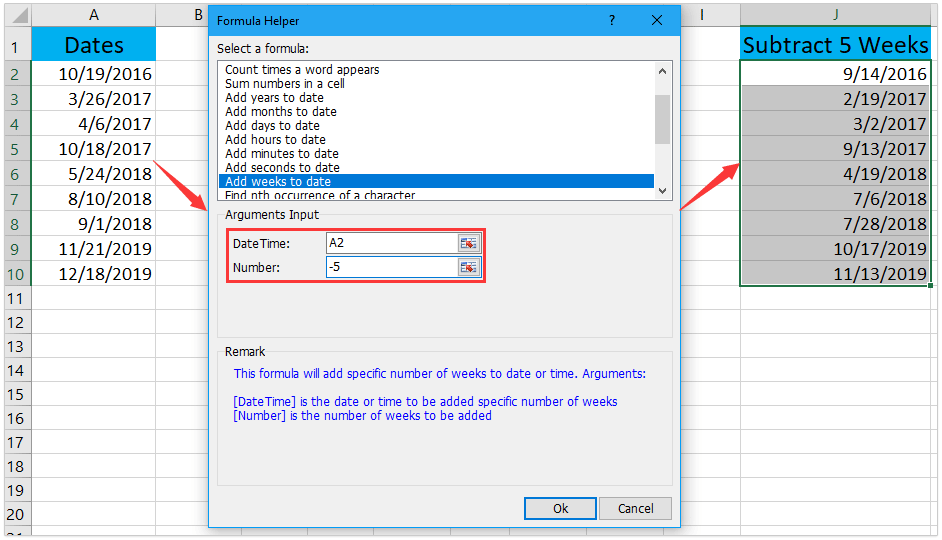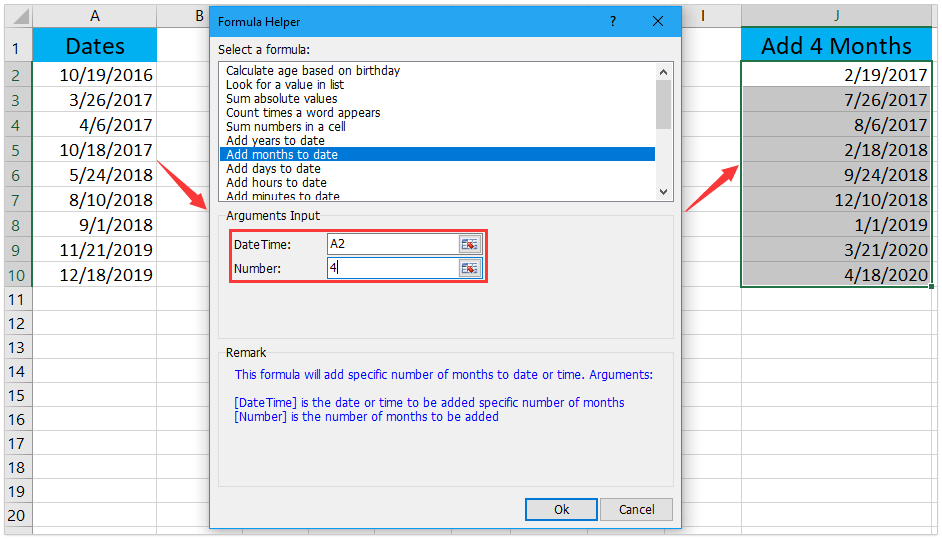Kutools for Excel’s Formula Helper will save Excel users from memorizing complicate formulas and lists most common-used formulas to help you quickly calculate and solve problems in Excel, says Add years / months / weeks / days to date, Add hours / minutes / seconds to date, etc. Have a Free Trial!

### with Kutools for Excel - never worry about job cutKutools for Excel brings 300 handy tools for 1500 work scenarios, helps you work faster and better than your colleagues, and easily win trust of your boss. You will be the last one in the list of layoffs, and easily maintain a stable and better life for your family!

• To be a master of Excel in 3 minutes, and easily win appreciation of others.
• Improve 80% work efficiency, solve your 80% problems in Excel, not work overtime.
• Speed up your work, save 2 hours every day to improve yourself and accompany family.
• Say goodbye to complicated formulas and VBA code, free up your memory.
• Reduce thousands of keyboard operations and mouse clicks, far away from mouse hand.
• Spend \$39.0, worth more than \$4000.0 training of others.
• Choice of
110,000+
highly effective people and 300+ famous companies, work stable in Excel.
• Full feature free trial 30-day, no credit card required.

No ratings yet. Be the first to rate!
This comment was minimized by the moderator on the site
1- 2year,4months,29days
and

2- 0year,9months,1days
how to calculate formula in Excel both 1 & 2 in same format like (3years 1 months 30days)
This comment was minimized by the moderator on the site
Hello, Nigam,
To solve your problem, the following User Defined Function may hep you:
``````Function CalculateDate(pRg As Range, pRg2 As Range)
On Error GoTo Err
Application.Volatile

Dim xRegEx As Object
Set xRegEx = CreateObject("VBSCRIPT.REGEXP")
y1 = 0
y2 = 0
y = 0
m1 = 0
m2 = 0
m = 0
d1 = 0
d2 = 0
d = 0
res = ""
With xRegEx
.Pattern = "\d+ ?year"
.Global = True
.IgnoreCase = True
End With
y1 = CInt(Replace(xRegEx.Execute(pRg.Value).Item(0), "year", ""))
y2 = CInt(Replace(xRegEx.Execute(pRg2.Value).Item(0), "year", ""))
xRegEx.Pattern = "\d+ ?months"
m1 = CInt(Replace(xRegEx.Execute(pRg.Value).Item(0), "months", ""))
m2 = CInt(Replace(xRegEx.Execute(pRg2.Value).Item(0), "months", ""))
xRegEx.Pattern = "\d+ ?days"
d1 = CInt(Replace(xRegEx.Execute(pRg.Value).Item(0), "days", ""))
d2 = CInt(Replace(xRegEx.Execute(pRg2.Value).Item(0), "days", ""))
d = d1 + d2
If d > 31 Then
d = d - 31
m = 1
End If
m = m + m1 + m2
If m > 12 Then
m = m - 12
y = 1
End If
y = y + y1 + y2
res = y & "year," & m & "months," & d & "days"
Err:
CalculateDate = res
End Function``````

After pasting this code, please apply this formula: =CalculateDate(A2,B2)

This comment was minimized by the moderator on the site
terima kasih, saya jadi mengetahui rumus menambahkan 1 bulan
This comment was minimized by the moderator on the site
Έχω σε κελιά το χρόνο υπηρεσίας υπαλλήλων
με τη μορφή "15χρ 00µη 17ηµ" (όλο σε ένα κελί).
Ξέρετε αν μπορώ να κάνω πράξεις με αυτό ???

π.χ. να Προσθέσω xxμη yyημ και να έχω 15χρ 00+xxµη 17+yyηµ
This comment was minimized by the moderator on the site
Hello, Ξερόλας!
Sorry, I can't understand your problem clearly, you can describe your problem in English.
Thank you!
This comment was minimized by the moderator on the site
Έχω σε κελιά το χρόνο υπηρεσίας υπαλλήλων
με τη μορφή "15χρ 00µη 17ηµ" (όλο σε ένα κελί).
Ξέρετε αν μπορώ να κάνω πράξεις με αυτό ???

π.χ. να Προσθέσω xxμη yyημ και να έχω 15χρ 00+xxµη 17+yyηµ
This comment was minimized by the moderator on the site
lo que requiero es sumar a una fecha solo los dias "4 martes" y "4 jueves"
This comment was minimized by the moderator on the site
hi, i need to substract dates formula of machine break down hoursi.e : machine breakdown started 1/1/2021  next cell 6:00 am job done 15/1/2021 4:00 PM
with minimum 10 hours each day = 150 hours(15 days)
can some one help me out on this formula..
This comment was minimized by the moderator on the site
How to add or subtract 5Y 8M 23D and 2Y 3M 12D in excel with formula
This comment was minimized by the moderator on the site
i need your help, i want to solve one question please tell me
one boy is going on leave from 26-06-2018 to 22-07-2018 for 27 days and his total monthly salary is 23968

if the month has 30 days then his salary will be per day 798.93 & if month has 31 days then 773.16

he was on leave in july 05 days(30 days in the month) and rest 22 dyas in july(31 days in the month)
23968/30*5+23968/31*44
i want then formula for this calculation in one cell please tell me as soon as possible because its urgent for me mail me on my personal mail
This comment was minimized by the moderator on the site
I used the DateDif to get the number of years, months, and days. Now I'm trying to total all of those years months and days. How to I do the total of all the columns for years months and days
This comment was minimized by the moderator on the site
I am trying to use the add month function, but I want the date to be based on the previous tab, so when I copy a new tab, it automatically updates the date based on adding a month to the previous tab's date. Any suggestions? Thank you - Brad
This comment was minimized by the moderator on the site